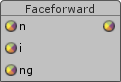## FANDOM

167 Pages## NameEdit

Faceforward - returns a normal as-is if a vertex's eye-space position vector points in the opposite direction of a geometric normal, otherwise return the negated version of the normal

## SynopsisEdit

```vector1 faceforward(vector1 N, vector1 I, vector1 Ng);
vector2 faceforward(vector2 N, vector2 I, vector2 Ng);
vector3 faceforward(vector3 N, vector3 I, vector3 Ng);
vector4 faceforward(vector4 N, vector4 I, vector4 Ng);
```

## ParametersEdit

N
Peturbed normal vector.
I
Incidence vector (typically a direction vector from the eye to a vertex).
Ng
Geometric normal vector (for some facet the peturbed normal belongs).

## DescriptionEdit

Returns a (peturbed) normal as-is if a vertex's eye-space position vector points in the opposite direction of a geometric normal, otherwise return the negated version of the (peturbed) normal

Mathematically, if the dot product of I and Ng is negative, N is returned unchanged; otherwise -N is returned.

This function is inspired by a RenderMan function of the same name though the RenderMan version has only two parameters.